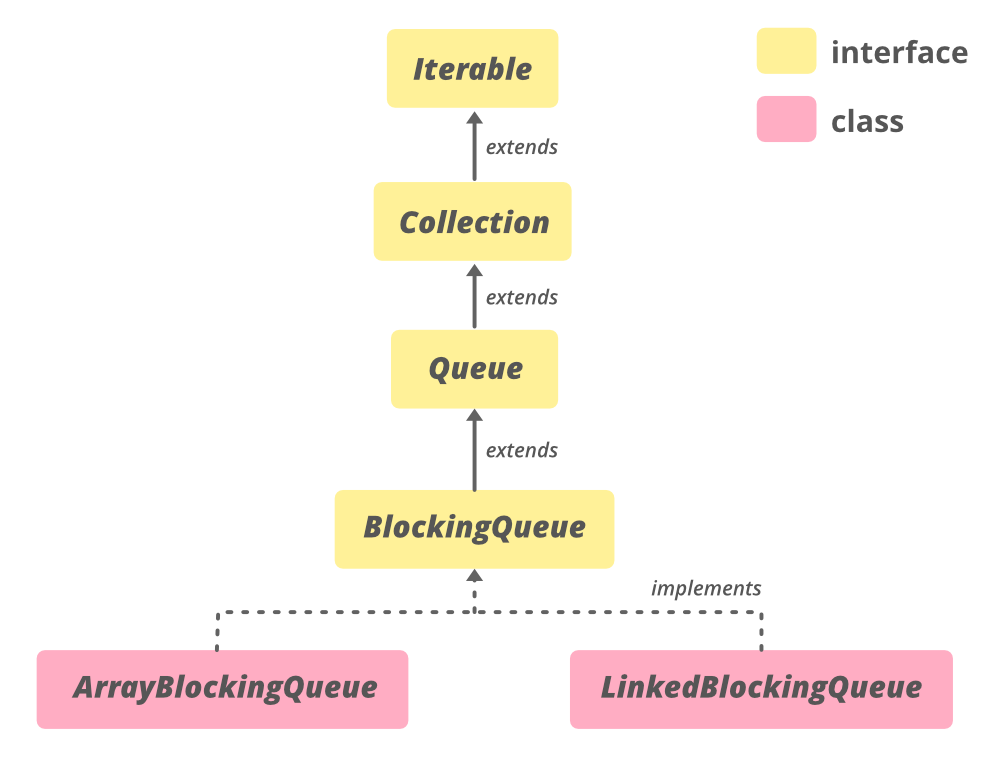Open In App

# ArrayBlockingQueue Class in Java

ArrayBlockingQueue class is a bounded blocking queue backed by an array. By bounded, it means that the size of the Queue is fixed. Once created, the capacity cannot be changed. Attempts to put an element into a full queue will result in the operation blocking. Similarly attempts to take an element from an empty queue will also be blocked. Boundness of the ArrayBlockingQueue can be achieved initially bypassing capacity as the parameter in the constructor of ArrayBlockingQueue. This queue orders elements FIFO (first-in-first-out). It means that the head of this queue is the oldest element of the elements present in this queue.

The tail of this queue is the newest element of the elements of this queue. The newly inserted elements are always inserted at the tail of the queue, and the queue retrieval operations obtain elements at the head of the queue.

This class and its iterator implement all of the optional methods of the Collection and Iterator interfaces. This class is a member of the Java Collections Framework.

The Hierarchy of ArrayBlockingQueueThis class extends AbstractQueue<E> and implements Serializable, Iterable<E>, Collection<E>, BlockingQueue<E>, Queue<E> interfaces.

Declaration

public class ArrayBlockingQueue<E> extends AbstractQueue<E> implements BlockingQueue<E>, Serializable

Here, E is the type of elements stored in the collection.

### Constructors of ArrayBlockingQueue

Here, capacity is the size of the array blocking queue.

1. ArrayBlockingQueue(int capacity): Creates an ArrayBlockingQueue with the given (fixed) capacity and default access policy.

ArrayBlockingQueue<E> abq = new ArrayBlockingQueue<E>(int capacity);

2. ArrayBlockingQueue(int capacity, boolean fair): Creates an ArrayBlockingQueue with the given (fixed) capacity and the specified access policy. If the fair value is if true then queue accesses for threads blocked on insertion or removal, are processed in FIFO order; if false the access order is unspecified.

ArrayBlockingQueue<E> abq = new ArrayBlockingQueue<E>(int capacity, boolean fair);

3. ArrayBlockingQueue(int capacity, boolean fair, Collection c): Creates an ArrayBlockingQueue with the given (fixed) capacity, the specified access policy and initially containing the elements of the given collection, added in traversal order of the collection’s iterator. If the fair value is if true then queue accesses for threads blocked on insertion or removal, are processed in FIFO order; if false the access order is unspecified.

ArrayBlockingQueue<E> abq = new ArrayBlockingQueue<E>(int capacity, boolean fair, Collection c);

Example:

## Java

 `// Java program to demonstrate ``// ArrayBlockingQueue(int initialCapacity)``// constructor`` ` `import` `java.util.concurrent.ArrayBlockingQueue;`` ` `public` `class` `ArrayBlockingQueueDemo {`` ` `    ``public` `static` `void` `main(String[] args)``    ``{``        ``// define capacity of ArrayBlockingQueue``        ``int` `capacity = ``15``;`` ` `        ``// create object of ArrayBlockingQueue``        ``// using ArrayBlockingQueue(int initialCapacity) constructor``        ``ArrayBlockingQueue abq = ``new` `ArrayBlockingQueue(capacity);`` ` `        ``// add  numbers``        ``abq.add(``1``);``        ``abq.add(``2``);``        ``abq.add(``3``);`` ` `        ``// print queue``        ``System.out.println(``"ArrayBlockingQueue:"` `+ abq);``    ``}``}`

Output:

`ArrayBlockingQueue:[1, 2, 3]`

### Basic Operations

The add(E e) method inserts the element passed as a parameter to the method at the tail of this queue. If adding the element exceeds the capacity of the queue then the method will throw an IllegalStateException. This method returns true if adding of the element is successful else it will throw an IllegalStateException.

## Java

 `// Java Program to Demonstrate adding``// elements to an ArrayBlockingQueue.`` ` `import` `java.util.concurrent.ArrayBlockingQueue;`` ` `public` `class` `AddingElementsExample {`` ` `    ``public` `static` `void` `main(String[] args)``    ``{``        ``// define capacity of ArrayBlockingQueue``        ``int` `capacity = ``15``;`` ` `        ``// create object of ArrayBlockingQueue``        ``ArrayBlockingQueue abq = ``new` `ArrayBlockingQueue(capacity);`` ` `        ``// add  numbers``        ``abq.add(``1``);``        ``abq.add(``2``);``        ``abq.add(``3``);`` ` `        ``// print queue``        ``System.out.println(``"ArrayBlockingQueue:"` `+ abq);``    ``}``}`

Output

`ArrayBlockingQueue:[1, 2, 3]`

2. Removing Elements

The remove(Object o) method removes a single instance of the specified element from this queue if it is present. We can say that method removes an element e such that o.equals(e) if this queue contains one or more such elements. Remove() method returns true if this queue contained the specified element which we want to remove.

## Java

 `// Java program to demonstrate removal of ``// elements from an AbstractQueue`` ` `import` `java.util.concurrent.ArrayBlockingQueue;`` ` `public` `class` `RemovingElementsExample {`` ` `    ``public` `static` `void` `main(String[] args)``    ``{``        ``// define capacity of ArrayBlockingQueue``        ``int` `capacity = ``15``;`` ` `        ``// create object of ArrayBlockingQueue``        ``ArrayBlockingQueue abq = ``new` `ArrayBlockingQueue(capacity);`` ` `        ``// add  numbers``        ``abq.add(``1``);``        ``abq.add(``2``);``        ``abq.add(``3``);`` ` `        ``// print queue``        ``System.out.println(``"ArrayBlockingQueue:"` `+ abq);`` ` `        ``// remove 223``        ``boolean` `response = abq.remove(``2``);`` ` `        ``// print Queue``        ``System.out.println(``"Removal of 2 :"` `+ response);`` ` `        ``// print Queue``        ``System.out.println(``"queue contains "` `+ abq);`` ` `        ``// remove all the elements``        ``abq.clear();`` ` `        ``// print queue``        ``System.out.println(``"ArrayBlockingQueue:"` `+ abq);``    ``}``}`

Output

```ArrayBlockingQueue:[1, 2, 3]
Removal of 2 :true
queue contains [1, 3]
ArrayBlockingQueue:[]```

3. Accessing Elements

The peek() method provided by the Queue interface is used to return the head of the queue. It retrieves but does not remove, the head of this queue. If the queue is empty then this method returns null.

## Java

 `// Java program to demonstrate accessing``// elements of ArrayBlockingQueue`` ` `import` `java.util.concurrent.ArrayBlockingQueue;`` ` `public` `class` `AccessingElementsExample {`` ` `    ``public` `static` `void` `main(String[] args)``    ``{`` ` `        ``// Define capacity of ArrayBlockingQueue``        ``int` `capacity = ``5``;`` ` `        ``// Create object of ArrayBlockingQueue``        ``ArrayBlockingQueue queue = ``new` `ArrayBlockingQueue(capacity);`` ` `        ``// Add element to ArrayBlockingQueue``        ``queue.add(``23``);``        ``queue.add(``32``);``        ``queue.add(``45``);``        ``queue.add(``12``);`` ` `        ``// Print queue after adding numbers``        ``System.out.println(``"After adding numbers queue is "``);``        ``System.out.println(queue);`` ` `        ``// Print head of queue using peek() method``        ``System.out.println(``"Head of queue "` `+ queue.peek());``    ``}``}`

Output

```After adding numbers queue is
[23, 32, 45, 12]

4. Traversing

The iterator() method of ArrayBlockingQueue class is used to returns an iterator of the same elements as this queue in a proper sequence. The elements returned from this method contains elements in order from first(head) to last(tail). The returned iterator is weakly consistent.

## Java

 `// Java Program to Demonstrate iterating``// over ArrayBlockingQueue.`` ` `import` `java.util.concurrent.ArrayBlockingQueue;``import` `java.util.*;`` ` `public` `class` `TraversingExample {`` ` `    ``public` `static` `void` `main(String[] args)``    ``{``        ``// Define capacity of ArrayBlockingQueue``        ``int` `capacity = ``5``;`` ` `        ``// Create object of ArrayBlockingQueue``        ``ArrayBlockingQueue queue = ``new` `ArrayBlockingQueue(capacity);`` ` `        ``// Add 5 elements to ArrayBlockingQueue``        ``queue.offer(``"User"``);``        ``queue.offer(``"Employee"``);``        ``queue.offer(``"Manager"``);``        ``queue.offer(``"Analyst"``);``        ``queue.offer(``"HR"``);`` ` `        ``// Print queue``        ``System.out.println(``"Queue is "` `+ queue);`` ` `        ``// Call iterator() method and Create an iterator``        ``Iterator iteratorValues = queue.iterator();`` ` `        ``// Print elements of iterator``        ``System.out.println(``"\nThe iterator values:"``);``        ``while` `(iteratorValues.hasNext()) {``            ``System.out.println(iteratorValues.next());``        ``}``    ``}``}`

Output

```Queue is [User, Employee, Manager, Analyst, HR]

The iterator values:
User
Employee
Manager
Analyst
HR```

### Methods of ArrayBlockingQueue

Here, E is the type of elements held in this collection

### Methods declared in interface java.util.Queue

Conclusion: ArrayBlockingQueue is generally used in a thread-safe environment where you want to block two or more operating on a single resource, allowing only one thread. Also, we can block a thread using the capacity bounding factor.